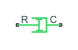# Rotational Damper

Viscous damper in mechanical rotational systems

## Library

Mechanical Rotational Elements

•## Description

The Rotational Damper block represents an ideal mechanical rotational viscous damper described with the following equations:

`$T=D·\omega$`
`$\omega ={\omega }_{R}-{\omega }_{C}$`

where

 T Torque transmitted through the damper D Damping (viscous friction) coefficient ω Relative angular velocity ωR, ωC Absolute angular velocities of terminals R and C, respectively

The block positive direction is from port R to port C. This means that the torque is positive if it acts in the direction from R to C.

### Variables

To set the priority and initial target values for the block variables prior to simulation, use the Initial Targets section in the block dialog box or Property Inspector. For more information, see Set Priority and Initial Target for Block Variables.

Nominal values provide a way to specify the expected magnitude of a variable in a model. Using system scaling based on nominal values increases the simulation robustness. Nominal values can come from different sources, one of which is the Nominal Values section in the block dialog box or Property Inspector. For more information, see Modify Nominal Values for a Block Variable.

## Parameters

Damping coefficient

Damping coefficient, defined by viscous friction. The default value is `0.001` N*m/(rad/s).

## Ports

The block has the following ports:

`R`

Mechanical rotational conserving port.

`C`

Mechanical rotational conserving port.

## Version History

Introduced in R2007a